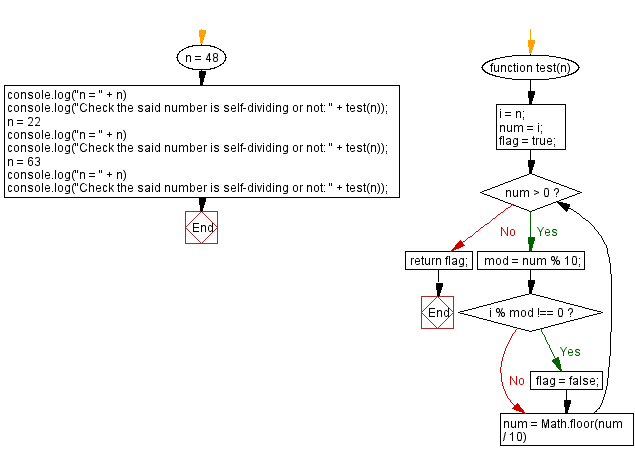# JavaScript: Check a given number is self-dividing or not

## JavaScript Math: Exercise-114 with Solution

Write a JavaScript program that checks if a given number is self-dividing.

In mathematics, a self-dividing number is one that can be divided by every digit (except digit zero) within it.

Test Data:
(48) -> true
(22) -> true
(63) -> false

Sample Solution:

HTML Code:

``````<!DOCTYPE html>
<html>
<meta charset="utf-8">
<title>JavaScript program to Check a given number is self-dividing or not</title>
<body>

</body>
</html>
```
```

JavaScript Code:

``````function test(n) {
i = n;
num = i;
flag = true;
while (num > 0) {

mod = num % 10;

if (i % mod !== 0) {
flag = false;
}

num = Math.floor(num / 10)
}
return flag;
}
n = 48
console.log("n = " + n)
console.log("Check the said number is self-dividing or not: " + test(n));
n = 22
console.log("n = " + n)
console.log("Check the said number is self-dividing or not: " + test(n));
n = 63
console.log("n = " + n)
console.log("Check the said number is self-dividing or not: " + test(n));
```
```

Sample Output:

```n = 48
Check the said number is self-dividing or not: true
n = 22
Check the said number is self-dividing or not: true
n = 63
Check the said number is self-dividing or not: false
```

Flowchart:Live Demo:

See the Pen javascript-math-exercise-114 by w3resource (@w3resource) on CodePen.

Improve this sample solution and post your code through Disqus

What is the difficulty level of this exercise?

Test your Programming skills with w3resource's quiz.

﻿